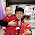## Monday, October 6, 2014

### Julia: The "Distributions" Package

This is a follow up to my post from a few days ago exploring random number generation in Julia's base system.  In this post I will explore the 'distributions' package.

You can find the excellent documentation on the "Distributions" package at:

# First let's set the current directory
cd("C:/Dropbox/Econometrics by Simulation/2014-10-October/")

# This post uses the following distributions
using Distributions

# I have got to say that I love the way Julia handles distributions
# as I discovered through this post.

# The Distributions package gives trenendous power to the user by
# providing a common framework to apply various function.

# For instance let's say you want to draw 10 draws from a Binomial(n=10, p=.25) distribution

rand(Binomial(10, .25), 1, 10)
#  4  3  0  5  1  3  5  2  2  1

# Looks pretty standard right? Well, what if we want the mean?

mean(Binomial(10, .25))
# 2.5

# mode, skewness, kurtosis, median?

a = Binomial(10, .25)
println("mode:", mode(a), " skewness:", skewness(a),
" kurtosis:", kurtosis(a), " median:", median(a))
# mode:3 skewness:0.3651483716701107 kurtosis:-0.06666666666666667 median:3

# Cool huh?

# Let's see how we can use Gadfly to easily plot some distributions:

# First we generate the plot (assign it to a 'p' for later drawing to disk)
#  In order to plot the different CDFs I will use an 'anonymous' function defined:
#  argument -> f(argument)
p=plot([x -> cdf(Normal(5,5^.5), x),
x -> cdf(Gamma(3,1), x),
x -> cdf(Exponential(2), x)]
, 0, 10)

# Write the graph to disk
draw(PNG("2014-10-06CDF.png", 24cm, 12cm), p)

g = plot([x -> pdf(Normal(5,2), x),
x -> pdf(Gamma(3,1), x),
x -> pdf(Exponential(2), x)]
, 0, 10)

draw(PNG("2014-10-06PDF.png", 24cm, 12cm), g)

1.1.